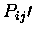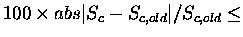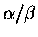Next: Summary of parameters for Up: Summary of STAMP parameters Previous: Summary of STAMP parameters

# Main program (STAMP)

The format for running STAMP is:

stamp -l <starting domain file> -s -o <output file> -P <parameter file>
-n <1 or 2 fits> -d <database file for scans>
-slide <slide value>
-pen1 <gap penatly 1> -pen2 <gap pentalty 2>
-prefix <output file prefix>
-V
-rough
-cut
-<parameter> <value>


If you have old STAMP parameter files, they can be read by using the command stamp -P <parameter file>. This means that the old file can be read in exactly the same way as for version 2.0.

In general, all commands can be specified by -<parameter> <value>. For example, -first_pairpen 0.5'. However, I have made some abbreviations for frequently used commands, these are:

-l <starting domain file>           same as -listfile <list file>
-o <output file>         same as -logfile <output file>
-n <1 or 2>              same as -npass <1 or 2>
-pen1 <gap penalty 1>    same as -first_pairpen <gap penalty 1>
-pen2 <gap penalty 2>    same as -second_pairpen <gap pentalty 2>
-prefix <output prefix>  same as -transprefix <output prefix>
-s                       same as -scan true
-d <database file>       same as -database <database file>
-slide <slide parameter> same as -scanslide <slide parameter>
-cut                     same as -co true
-rough                   same as -roughfit true


Default parameters are always looked for in the file STAMPDIR/stamp.defaults. You can personalise this is as you like, but I would recommend using the defaults, unless you have a thorough understnading of the method. The values described below were essentially chosen to mimick the successful and well-tested parameters .

I would recommend using the command line parameters. The commands, and their arguments are given below. The command line parameters are case insensitive. To use a parameter one need only type -<parameter> <value>' or use on of the short forms listed above.

STAMP can also be supplied with a parameter file. Parameters in a parameter file can be supplied in the format:

<Parameter> <Value> <Optional Comments> [return]


eg.

PAIRWISE Yes   Perform pairwise calculations
E1 3.8
E2 3.8
CUTOFF 4.5


Since the program is written in C, the input is read in an open format. Generally, data are expected to be separated by spaces or return characters. The number and position of spaces, tabs and returns generally should not matter with the exception of PDB format, which is read as the fixed format described in the brookhaven documentation.

The possible parameters are listed below. Strings, characters, floats and integers are as expected (though strings may not contain spaces). Boolean variables may be set by any of the following:

TRUE  == TRUE, True, T, true, Yes, YES, yes, Y, 1
FALSE == FALSE, False, F, false, No, NO, n, 0


LOGFILE <string>
This is the file into which the log is to be written. If stdout' is supplied then the information is written to the standard output.
Default LOGFILE = stdout

LISTFILE <string> (or -l <string>' or -f <string>')
This is the name of a file that contains the location and description of the domains to be analysed and, if desired, an initial transformation.
Default LISTFILE = domain.list

SECTYPE <integer>
This must be set to 0 (no secondary structure assignment) or to one of the following values:

SECTYPE = 1 Kabsch and Sander's DSSP output. This program, which calculates secondary structure based on hydrogen bonding criteria  is available from the EMBL fileserver.
SECTYPE = 2 Secondary structure summary format. A string of residue by residue secondary structure assignments for each domain is to be read in from SECFILE in the format specified in the previous chapter.

Note that it is not possible to mix assignments. This is probably not a very realistic thing to do anyway, since assignments can differ substantially. If you really want to do this, then the only possible way is to set SECTYPE = 3, and define each secondary structure independently in SECFILE.
Default SECTYPE = 1 (for DSSP).

SECFILE <string>
The file from which user specified secondary structure assignments are to be read (ie. SECTYPE = 2 only).
Default SECFILE = stamp.sec

PAIRWISE <boolean>
If TRUE, then pairwise comparisons are to be performed for each possible pair of domains described in LISTFILE. A matrix of pairwise (Sc) scores will be output (to MATFILE).
Default PAIRWISE = TRUE

N.B. Many of the following parameters also apply to TREEWISE and SCAN comparisons. For clarity they are discussed here in the PAIRWISE comparison context.

NPASS <1 or 2> (or -n <1 or 2>')
Whether one or two fits are to be performed. The idea is that the initial fit can be used with a conformation biased set of parameters to improve the initial fit prior to fitting using distance and conformation parameters. The parameters described below are called first_' and second_' accordingly. When NPASS = 1, then only the second_' (or unprefixed) parameters are used. Default NPASS = 1

SW <0 or 1>
If set to 0, then the entire M x N matrix will be calculated and used during the Smith Waterman path finding routine. If set to 1, then a corner cutting routine will be used (to save time). Note that corner cutting will nullify many of the parameters specified in , and is only recommended for SCAN mode. Accordingly, corner cutting parameters are specified below (after SCAN).

PAIRPEN <float> (or -pen1 <float>'/ -pen2 <float>')
(first_PAIRPEN)
(second_PAIRPEN)
Smith-Waterman gap penalty to be used during the fitting. second_PAIRPEN and PAIRPEN are equivalent. (PAIRPEN is also relevant to treewise fitting)
Defaults PAIRPEN = second_PAIRPEN = 0.0 first_PAIRPEN = 0.0

E1 <float>
E2 <float>
(first_E1,first_E2)
(second_E2,second_E2)

Rossmann and Argos parameters to be used during the fitting. Rossmann and Argos suggested that E1 = E2 = 3.8 lead to good superimpositions, and further suggested that E1 = 20.0 and E2 = 3.8 would relax the distance requirement, and allow poor initial superimpositions to be improved. The defaults are defined accordingly.
Defaults:
E1 = second_E1 = 3.8
E2 = second_E2 = 3.8
first_E1 = 20.0
first_E2 = 3.8

I would not recommend modifying these parameters, since I really don't know what changing them will do. If it ain't broke, don't fix it as my father would say.

NA <float>
NB <float>
NASD <float>
NBSD <float>
NSD <float>
NMEAN <float>

Parameters used to defineand Sc values. These are defined in . I wouldn't change these.

Defaults:
NA = -0.9497
NB = 0.6859
NASD = -0.4743
NBSD = 0.01522
NMEAN = 0.02
NSD = 0.1

CUTOFF <float>
(first_CUTOFF)
(second_CUTOFF)
This is the minimumvalue allowed for atoms to be used for a least squares fit. Equivalences above this value will be used to determine a transformation and RMS deviation.
Defaults:
CUTOFF = second_CUTOFF = 4.5
first_CUTOFF = 1.0

PAIRALIGN <boolean>
If true, then each final pairwise alignment will be output to the log file.
Default PAIRALIGN = FALSE

COLUMNS <integer>
Number of sequence positions to be displayed per line when either PAIRALIGN, SCANALIN or TREEALIGN is set to TRUE.
Default COLUMNS = 80

SCORETOL <float>
This is the percent Sc difference that will result in convergence being reached. In other words, ifSCORETOL then the fitting will be considered done.
Default SCORETOL = 1.0

MAXPITER <integer>
The maximum number of iterations allowed during the pairwise comparisons. This prevents a particular fit, which jumps between two values rather than converging, from lasting indefinitely.
Default MAXPITER = 10

MATFILE <string>
This is the file which contains an upper diagonal matrix consisting of the pairwise Scores (either 1/RMS, or Sc) for each comparison. It may then be used to derive a tree, if desired, for treewise analysis.
Default MATFILE = <stamp_prefix>.mat

ROUGHFIT <boolean> (or -rough' to set to TRUE)
If set to TRUE, then an initial rough superimposition will be performed by aligning the N-terminal ends of the sequences and fitting on whatever atoms this process equivalences. Probably this is too crude for structures that differ quite a bit, but if they are very similar, one can use this to avoid having to perform a multiple sequence alignment.

TREEWISE <boolean>
If TRUE, then a treewise comparison is performed by following a derived hierarchy. Reads in the matrix file specified (either created by PAIRWISE or some other method), derives a tree (dendrogram), and does a tree-based alignment.
Default TREEWISE = TRUE

TREEPEN <float>
(first_TREEPEN)
(second_TREEPEN)
Value subtracted from thematrix at positions where a residue is to be aligned with a gap. For details see .
Defaults TREEPEN = second_TREEPEN = 0.0 first_TREEPEN = 0.0

MAXTITER <int>
As for MAXPITER, but applied to the treewise case.
Default MAXPITER = 10

TREEALIGN <boolean>
As for PAIRALIGN, only for treewise comparisons.
Default TREEALIGN = TRUE

STAMPPREFIX <string> (or -prefix <string>')
This is the name of the family of files that will be produced from a multiple alignment. The files will be named STAMPPREFIX.<N>, where N is the number of the cluster after which the alignment has been derived. There are always one fewer clusters than their are domains being compared.
Default STAMPPREFIX = stamp_trans'

SCAN <boolean> (or simply -s' to set true)
If TRUE, then SCAN mode is selected. TREEWISE and PAIRWISE are set to FALSE. The first domain described in LISTFILE (the query) is used to scan all the domains listed in DATABASE. The parameters for scanning are described below. The output of a SCAN run appears in the file called STAMPPREFIX.scan.
Default SCAN = FALSE

DATABASE <string> (or -d <string>)
The list of domains to be compared with the query during a scan.
Default DATABASE = domain.database

MAXSITER <int>
As for MAXPITER and MAXTITER, but for scanning. Equivalent within the program to MAXPITER.
Default MAXSITER = 10

SCANALIGN <boolean>
As for PAIRALIGN and TREEALIGN, but for scanning. Equivalent within the program to MAXPITER.
Default SCANALIGN = FALSE

SCANSCORE <integer>
Specifies how the Sc value is to be calculated. This depends on the particular application. The values are described in the first chapter.

As a general rule of thumb, use SCANSCORE=6 for large database scans, when you are scanning with a small domain, and wishing to find all examples of this domain - even within large structures. Use SCANSCORE=1 when you wish to obtain a set of transformations for a set of domains which you know are similar (and have defined fairly precisely as domains rather than the larger structure that they may be a part of).
Default SCANSCORE = 6

If set to TRUE, then the program will skip over all hits. In other words, if a similarity is found with a particular starting fit position, then the next fit position will be the last residue of the similar region. This is not always desireable, since there can be more than one hit within repetetive structures, such asbarrels.
Default SKIPAHED = TRUE

OPD <boolean>
Means One Per Domain''. When the first hit for a domain is found during a SCAN (i.e. with Sc above SCANCUT), the rest of the comparisons involving that domain are skipped. Means that multiple matches involving the probe and database structures will be missed.
Default OPD = FALSE

SCANCUT <float>
If SCANMODE = 1, then Sc must be >= SCANCUT in order for a transformation to be output.
Default SCANCUT = 2.0

SCANSLIDE <integer> (or -slide <integer>') This is the number of residues that a query sequence is slid along a database sequence to derive each initial superimposition. Initially, the N-terminus of the query is aligned to the 1st residue of the databse, once this fit has been performed and refined, and tested for good structural similarity, the N-terminus is aligned with the 1+<SCANSLIDE>th position, and the process repeated until the end of the database sequence has been reached.
Default SCANSLIDE = 5

SCANTRUNC <boolean>
If TRUE, then sequences from DATABASE that are more than SCANTRUNCFACTOR x the length of the query sequence are truncated to this size. This saves a lot of CPU time, as comparisons between things that are vastly different in size are largely meaningless. Moreover, since most scans will be done with discrete domains, then this allows separate domains in large proteins to be compared to the query separately.
Default SCANTRUNC = TRUE

SCANTRUNCFACTOR <float>
The largest size of sequence which may be compared to the query sequence (expressed as SCANTRUNCFACTOR x query sequence length). Structures in the DATABASE that are larger than this will be truncated to this size if SCANTRUNC = TRUE.
Default SCANTRUNCFACTOR = 2.0

SLOWSCAN <boolean>
If set to TRUE, then the SLOW method of getting the initial fits for scanning will be used (See chapter 1).
Default SLOWSCAN = FALSE

MIN_FRAC <float>
This is the minimum ratio of database length/query length to be allowed. In other words, if a database structure is too small (ie. if databaselength/query length < MIN_FRAC), then the comparison will be skipped. Whether to use this or not depends on whether or not one is interested in sub alignments where only a part of the query structure is used. The default implies that all comparisons will be performed.
Default MIN_FRAC = 0.001

SECSCREEN <boolean>
If TRUE, then an initial comparison between query and DATABASE secondary structure assignments (if available) is performed. A secondary structure distance is defined by:where Qh and Qb are the percent of Helix and Beta structure in the query, and Dh and Db are the same for the database sequence. If Dist is larger than a threshold (SECSCREENMAX) then the comparison will be ignored.
Default SECSCREEN = true

SECSCREENMAX <float>
This is the maximum value of Dist (above) tolerated. If Dist is larger than SECSCREENMAX then the comparison is ignored. For screening to be effective, it is important that secondary structure assignments are accurate (preferably done using the same program).
Default SECSCREENMAX = 60.0 (this is very lenient; 40 is usually safe)

CCFACTOR <float>
Corner cutting factor. This is approximately the maximum number of gaps to be tolerated in any pairwise comparison. Only used if SW = 1. For a more detailed explanation, refer to  (pp 279 - 281).
Default CCFACTOR = 30.0

If TRUE, then the difference between query and database sequence lengths will be added to CCFACTOR. Probably this is only realistic when SCANTRUNC is set TRUE.

PRECISION <integer>
Since STAMP works as much as possible with integers, this is what all floating point values are multiplied by during conversion. A value of 1000 has never presented us with any problems.
Default PRECISION = 1000

MAX_SEQ_LEN <integer>
The maximum length of alignment tolerated. The program ought to inform you when this value is surpassed.
Default MAX_SEQ_LEN = 1500Next: Summary of parameters for Up: Summary of STAMP parameters Previous: Summary of STAMP parameters
Geoff Barton
1999-04-16